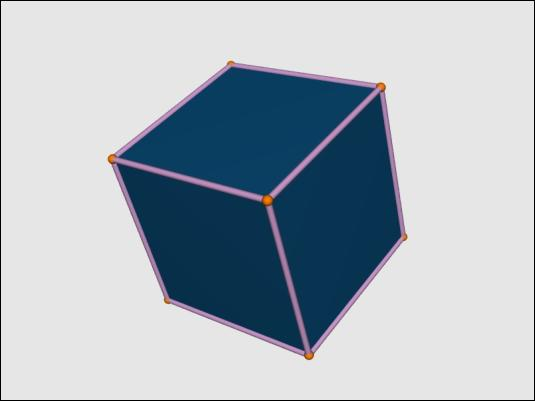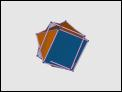Description : The -R option specifies a rotation about the origin. If the argument is six values this is a rotation of a 'from' vector (the first three numbers) onto a 'to' vector (the second set of three numbers) about a axis perpendicular to the two vectors. In this example aligning a vertex of a cube with the z-axis the rotations does not nicely align the cube with the x and y axes, for that see the 12 parameter rotation. View this model with the command `off_trans -R 1,1,1,0,0,1 cube | antiview`previous thumbnails Image List next